Anúncio

# ect292 nanoelectronics

19 de Jan de 2022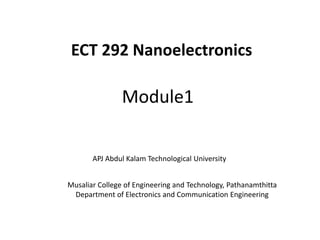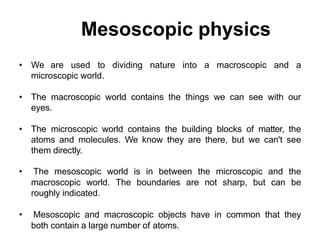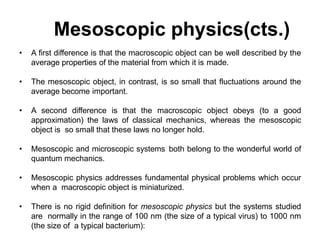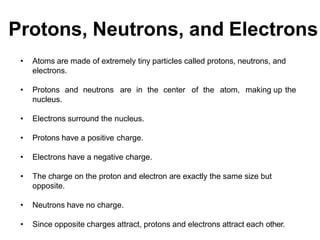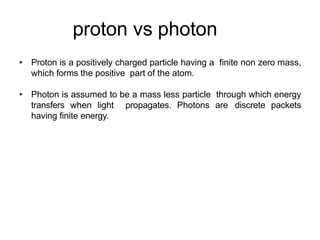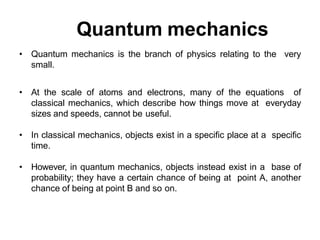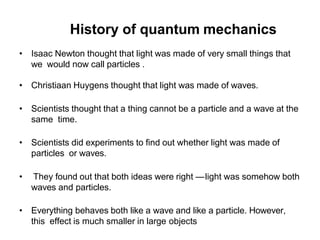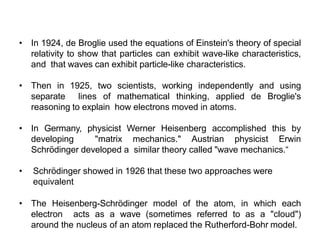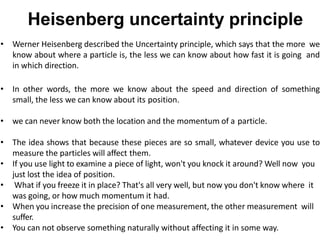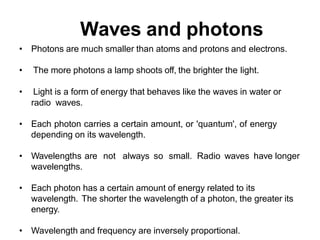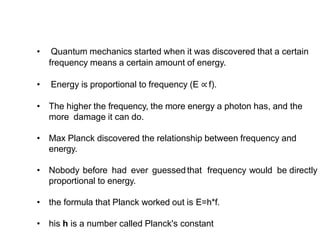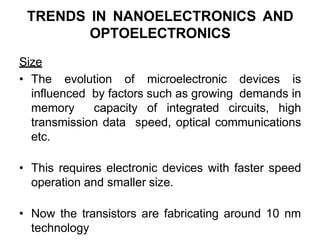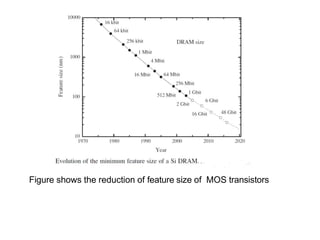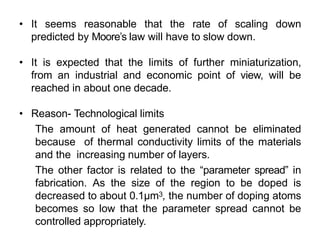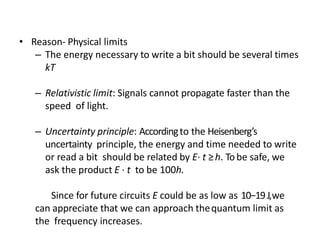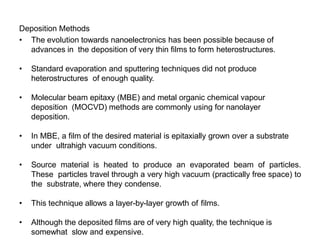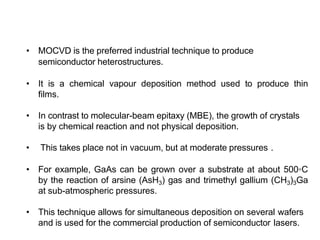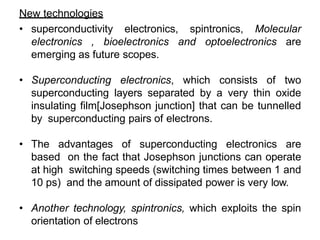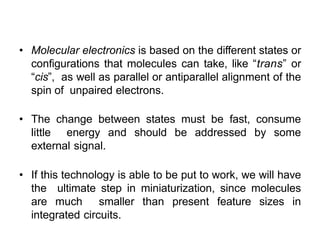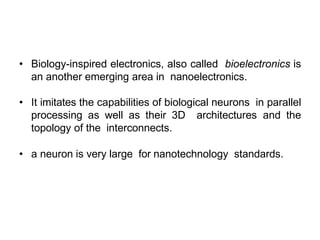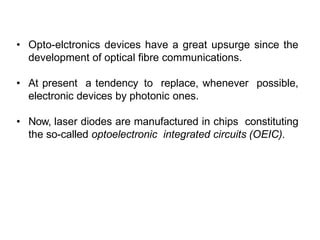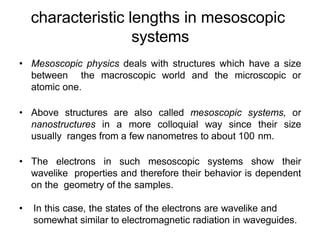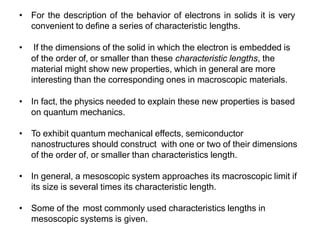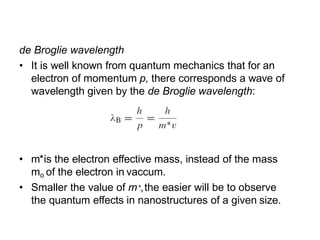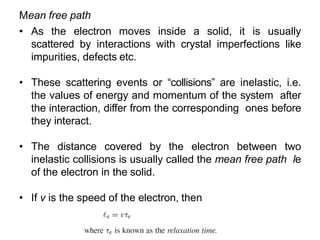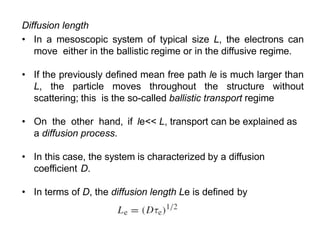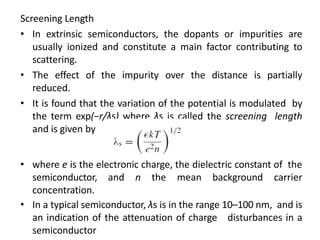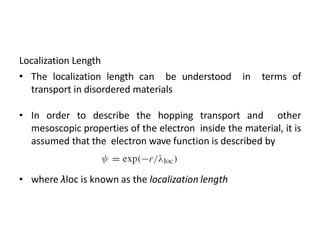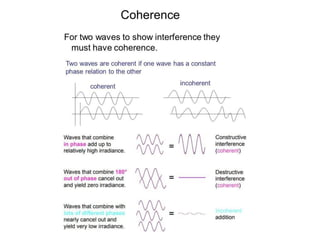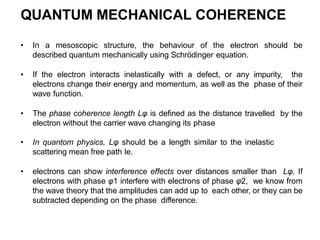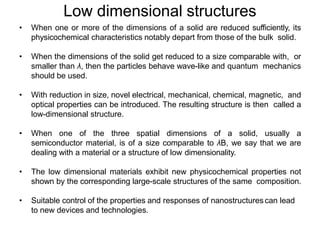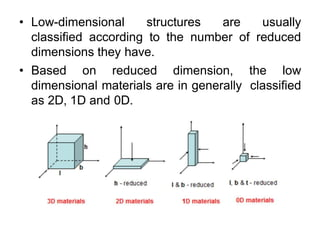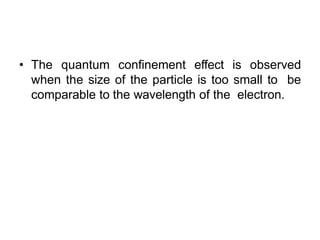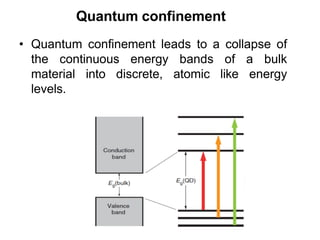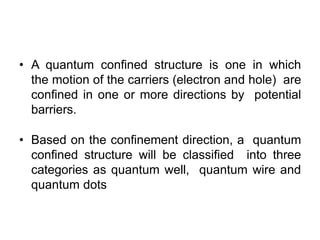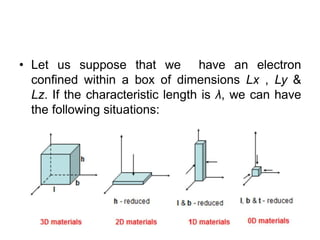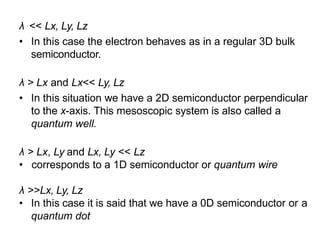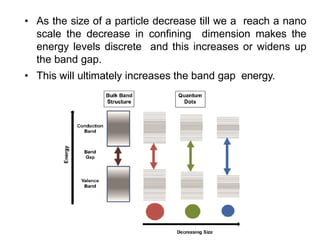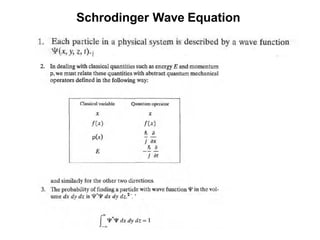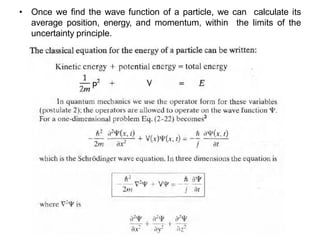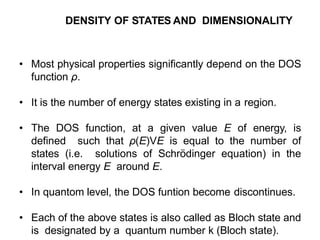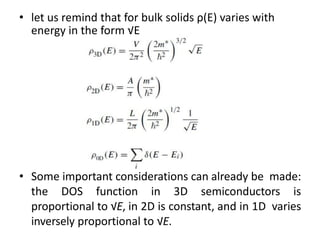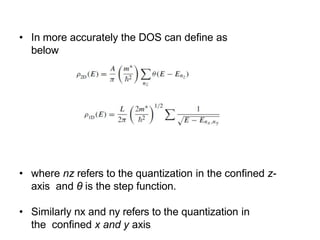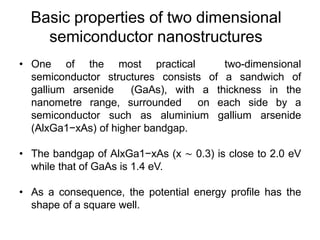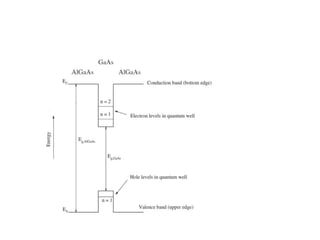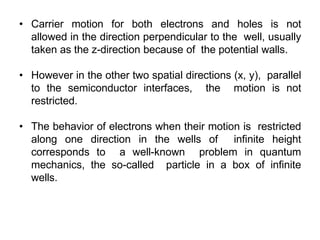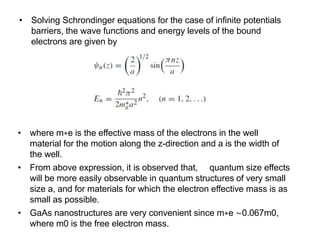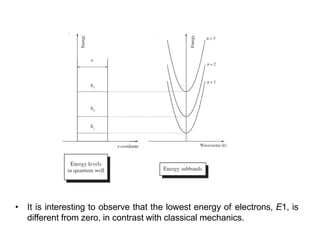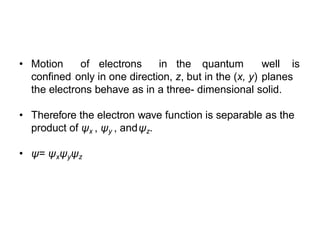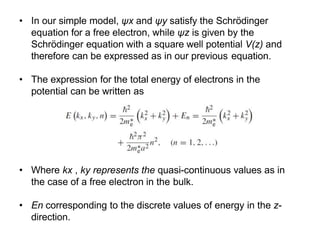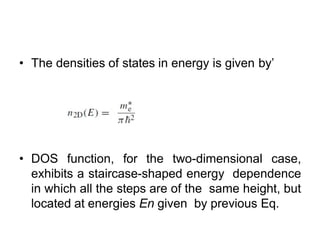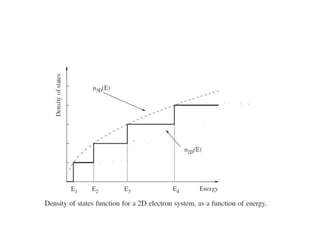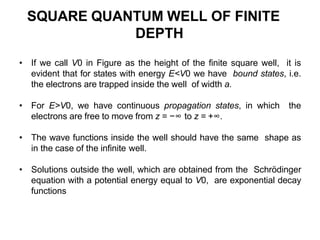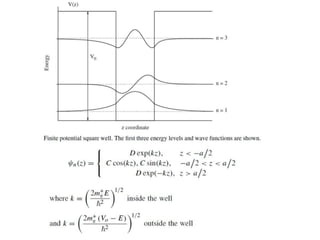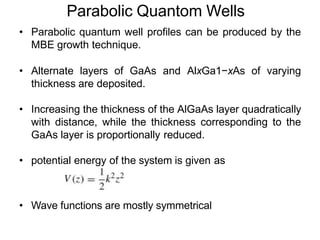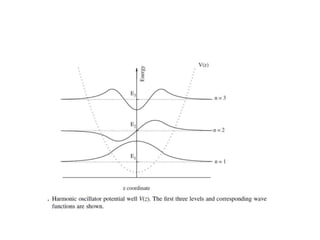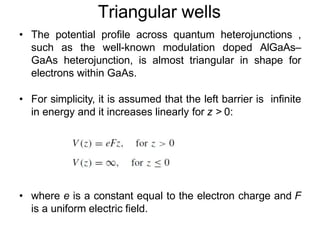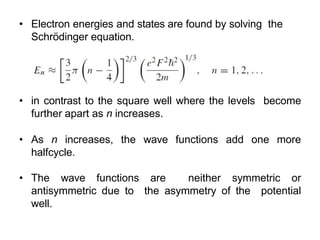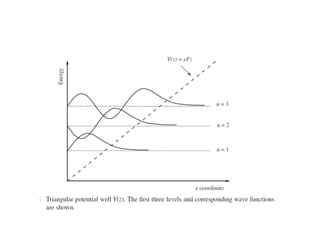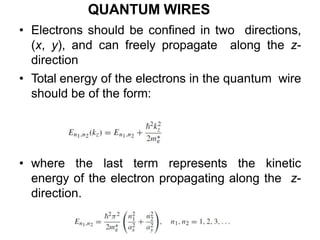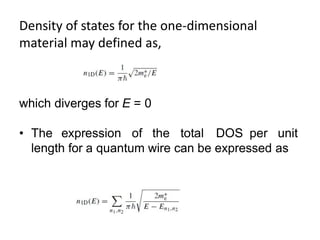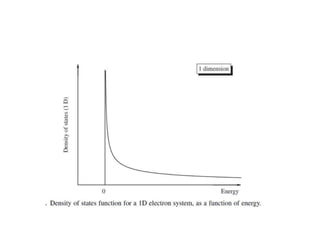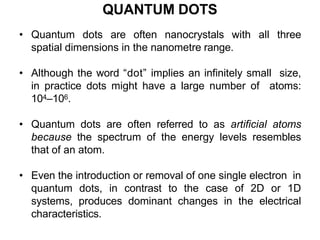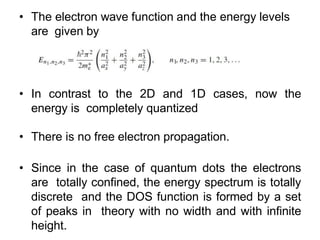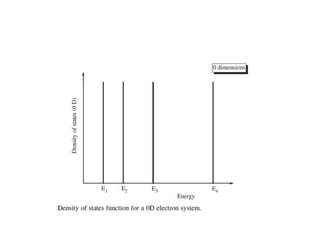1 de 66
Anúncio

### ect292 nanoelectronics

1. Module1 Musaliar College of Engineering and Technology, Pathanamthitta Department of Electronics and Communication Engineering ECT 292 Nanoelectronics APJ Abdul Kalam Technological University
2. Mesoscopic physics • We are used to dividing nature into a macroscopic and a microscopic world. • The macroscopic world contains the things we can see with our eyes. • The microscopic world contains the building blocks of matter, the atoms and molecules. We know they are there, but we can't see them directly. • The mesoscopic world is in between the microscopic and the macroscopic world. The boundaries are not sharp, but can be roughly indicated. • Mesoscopic and macroscopic objects have in common that they both contain a large number of atoms.
3. Mesoscopic physics(cts.) • A first difference is that the macroscopic object can be well described by the average properties of the material from which it is made. • The mesoscopic object, in contrast, is so small that fluctuations around the average become important. • A second difference is that the macroscopic object obeys (to a good approximation) the laws of classical mechanics, whereas the mesoscopic object is so small that these laws no longer hold. • Mesoscopic and microscopic systems both belong to the wonderful world of quantum mechanics. • Mesoscopic physics addresses fundamental physical problems which occur when a macroscopic object is miniaturized. • There is no rigid definition for mesoscopic physics but the systems studied are normally in the range of 100 nm (the size of a typical virus) to 1000 nm (the size of a typical bacterium):
4. Protons, Neutrons, and Electrons • Atoms are made of extremely tiny particles called protons, neutrons, and electrons. • Protons and neutrons are in the center of the atom, making up the nucleus. • Electrons surround the nucleus. • Protons have a positive charge. • Electrons have a negative charge. • The charge on the proton and electron are exactly the same size but opposite. • Neutrons have no charge. • Since opposite charges attract, protons and electrons attract each other.
5. proton vs photon • Proton is a positively charged particle having a finite non zero mass, which forms the positive part of the atom. • Photon is assumed to be a mass less particle through which energy transfers when light propagates. Photons are discrete packets having finite energy.
6. Quantum mechanics • Quantum mechanics is the branch of physics relating to the very small. • At the scale of atoms and electrons, many of the equations of classical mechanics, which describe how things move at everyday sizes and speeds, cannot be useful. • In classical mechanics, objects exist in a specific place at a specific time. • However, in quantum mechanics, objects instead exist in a base of probability; they have a certain chance of being at point A, another chance of being at point B and so on.
7. History of quantum mechanics • Isaac Newton thought that light was made of very small things that we would now call particles . • Christiaan Huygens thought that light was made of waves. • Scientists thought that a thing cannot be a particle and a wave at the same time. • Scientists did experiments to find out whether light was made of particles or waves. • They found out that both ideas were right —light was somehow both waves and particles. • Everything behaves both like a wave and like a particle. However, this effect is much smaller in large objects
8. • In 1924, de Broglie used the equations of Einstein's theory of special relativity to show that particles can exhibit wave-like characteristics, and that waves can exhibit particle-like characteristics. • Then in 1925, two scientists, working independently and using separate lines of mathematical thinking, applied de Broglie's reasoning to explain how electrons moved in atoms. • In Germany, physicist Werner Heisenberg accomplished this by developing "matrix mechanics." Austrian physicist Erwin Schrödinger developed a similar theory called "wave mechanics.“ • Schrödinger showed in 1926 that these two approaches were equivalent • The Heisenberg-Schrödinger model of the atom, in which each electron acts as a wave (sometimes referred to as a "cloud") around the nucleus of an atom replaced the Rutherford-Bohr model.
9. Heisenberg uncertainty principle • Werner Heisenberg described the Uncertainty principle, which says that the more we know about where a particle is, the less we can know about how fast it is going and in which direction. • In other words, the more we know about the speed and direction of something small, the less we can know about its position. • we can never know both the location and the momentum of a particle. • The idea shows that because these pieces are so small, whatever device you use to measure the particles will affect them. • If you use light to examine a piece of light, won't you knock it around? Well now you just lost the idea of position. • What if you freeze it in place? That's all very well, but now you don't know where it was going, or how much momentum it had. • When you increase the precision of one measurement, the other measurement will suffer. • You can not observe something naturally without affecting it in some way.
10. Waves and photons • Photons are much smaller than atoms and protons and electrons. • The more photons a lamp shoots off, the brighter the light. • Light is a form of energy that behaves like the waves in water or radio waves. • Each photon carries a certain amount, or 'quantum', of energy depending on its wavelength. • Wavelengths are not always so small. Radio waves have longer wavelengths. • Each photon has a certain amount of energy related to its wavelength. The shorter the wavelength of a photon, the greater its energy. • Wavelength and frequency are inversely proportional.
11. • Quantum mechanics started when it was discovered that a certain frequency means a certain amount of energy. • Energy is proportional to frequency (E ∝ f). • The higher the frequency, the more energy a photon has, and the more damage it can do. • Max Planck discovered the relationship between frequency and energy. • Nobody before had ever guessedthat frequency would be directly proportional to energy. • the formula that Planck worked out is E=h*f. • his h is a number called Planck's constant
12. TRENDS IN NANOELECTRONICS AND OPTOELECTRONICS Size • The evolution of microelectronic devices is influenced by factors such as growing demands in memory capacity of integrated circuits, high transmission data speed, optical communications etc. • This requires electronic devices with faster speed operation and smaller size. • Now the transistors are fabricating around 10 nm technology
13. Figure shows the reduction of feature size of MOS transistors
14. • It seems reasonable that the rate of scaling down predicted by Moore’s law will have to slow down. • It is expected that the limits of further miniaturization, from an industrial and economic point of view, will be reached in about one decade. • Reason- Technological limits The amount of heat generated cannot be eliminated because of thermal conductivity limits of the materials and the increasing number of layers. The other factor is related to the “parameter spread” in fabrication. As the size of the region to be doped is decreased to about 0.1μm3, the number of doping atoms becomes so low that the parameter spread cannot be controlled appropriately.
15. • Reason- Physical limits – The energy necessary to write a bit should be several times kT – Relativistic limit: Signals cannot propagate faster than the speed of light. – Uncertainty principle: Accordingto the Heisenberg’s uncertainty principle, the energy and time needed to write or read a bit should be related by E· t ≥h. Tobe safe, we ask the product E · t to be 100h. Since for future circuits E could be as low as 10−19J,we can appreciate that we can approach thequantum limit as the frequency increases.
16. Deposition Methods • The evolution towards nanoelectronics has been possible because of advances in the deposition of very thin films to form heterostructures. • Standard evaporation and sputtering techniques did not produce heterostructures of enough quality. • Molecular beam epitaxy (MBE) and metal organic chemical vapour deposition (MOCVD) methods are commonly using for nanolayer deposition. • In MBE, a film of the desired material is epitaxially grown over a substrate under ultrahigh vacuum conditions. • Source material is heated to produce an evaporated beam of particles. These particles travel through a very high vacuum (practically free space) to the substrate, where they condense. • This technique allows a layer-by-layer growth of films. • Although the deposited films are of very high quality, the technique is somewhat slow and expensive.
17. • MOCVD is the preferred industrial technique to produce semiconductor heterostructures. • It is a chemical vapour deposition method used to produce thin films. • In contrast to molecular-beam epitaxy (MBE), the growth of crystals is by chemical reaction and not physical deposition. • This takes place not in vacuum, but at moderate pressures . • For example, GaAs can be grown over a substrate at about 500◦C by the reaction of arsine (AsH3) gas and trimethyl gallium (CH3)3Ga at sub-atmospheric pressures. • This technique allows for simultaneous deposition on several wafers and is used for the commercial production of semiconductor lasers.
18. New technologies • superconductivity electronics, spintronics, Molecular electronics , bioelectronics and optoelectronics are emerging as future scopes. • Superconducting electronics, which consists of two superconducting layers separated by a very thin oxide insulating film[Josephson junction] that can be tunnelled by superconducting pairs of electrons. • The advantages of superconducting electronics are based on the fact that Josephson junctions can operate at high switching speeds (switching times between 1 and 10 ps) and the amount of dissipated power is very low. • Another technology, spintronics, which exploits the spin orientation of electrons
19. • Molecular electronics is based on the different states or configurations that molecules can take, like “trans” or “cis”, as well as parallel or antiparallel alignment of the spin of unpaired electrons. • The change between states must be fast, consume little energy and should be addressed by some external signal. • If this technology is able to be put to work, we will have the ultimate step in miniaturization, since molecules are much smaller than present feature sizes in integrated circuits.
20. • Biology-inspired electronics, also called bioelectronics is an another emerging area in nanoelectronics. • It imitates the capabilities of biological neurons in parallel processing as well as their 3D architectures and the topology of the interconnects. • a neuron is very large for nanotechnology standards.
21. • Opto-elctronics devices have a great upsurge since the development of optical fibre communications. • At present a tendency to replace, whenever possible, electronic devices by photonic ones. • Now, laser diodes are manufactured in chips constituting the so-called optoelectronic integrated circuits (OEIC).
22. characteristic lengths in mesoscopic systems • Mesoscopic physics deals with structures which have a size between the macroscopic world and the microscopic or atomic one. • Above structures are also called mesoscopic systems, or nanostructures in a more colloquial way since their size usually ranges from a few nanometres to about 100 nm. • The electrons in such mesoscopic systems show their wavelike properties and therefore their behavior is dependent on the geometry of the samples. • In this case, the states of the electrons are wavelike and somewhat similar to electromagnetic radiation in waveguides.
23. • For the description of the behavior of electrons in solids it is very convenient to define a series of characteristic lengths. • If the dimensions of the solid in which the electron is embedded is of the order of, or smaller than these characteristic lengths, the material might show new properties, which in general are more interesting than the corresponding ones in macroscopic materials. • In fact, the physics needed to explain these new properties is based on quantum mechanics. • To exhibit quantum mechanical effects, semiconductor nanostructures should construct with one or two of their dimensions of the order of, or smaller than characteristics length. • In general, a mesoscopic system approaches its macroscopic limit if its size is several times its characteristic length. • Some of the most commonly used characteristics lengths in mesoscopic systems is given.
24. de Broglie wavelength • It is well known from quantum mechanics that for an electron of momentum p, there corresponds a wave of wavelength given by the de Broglie wavelength: • m*is the electron effective mass, instead of the mass mo of the electron in vaccum. • Smaller the value of m∗,the easier will be to observe the quantum effects in nanostructures of a given size.
25. Mean free path • As the electron moves inside a solid, it is usually scattered by interactions with crystal imperfections like impurities, defects etc. • These scattering events or “collisions” are inelastic, i.e. the values of energy and momentum of the system after the interaction, differ from the corresponding ones before they interact. • The distance covered by the electron between two inelastic collisions is usually called the mean free path le of the electron in the solid. • If v is the speed of the electron, then
26. Diffusion length • In a mesoscopic system of typical size L, the electrons can move either in the ballistic regime or in the diffusive regime. • If the previously defined mean free path le is much larger than L, the particle moves throughout the structure without scattering; this is the so-called ballistic transport regime • On the other hand, if le<< L, transport can be explained as a diffusion process. • In this case, the system is characterized by a diffusion coefficient D. • In terms of D, the diffusion length Le is defined by
27. Screening Length • In extrinsic semiconductors, the dopants or impurities are usually ionized and constitute a main factor contributing to scattering. • The effect of the impurity over the distance is partially reduced. • It is found that the variation of the potential is modulated by the term exp(−r/λs) where λs is called the screening length and is given by • where e is the electronic charge, the dielectric constant of the semiconductor, and n the mean background carrier concentration. • In a typical semiconductor, λs is in the range 10–100 nm, and is an indication of the attenuation of charge disturbances in a semiconductor
28. Localization Length • The localization length can be understood in terms of transport in disordered materials • In order to describe the hopping transport and other mesoscopic properties of the electron inside the material, it is assumed that the electron wave function is described by • where λloc is known as the localization length
29. QUANTUM MECHANICAL COHERENCE • In a mesoscopic structure, the behaviour of the electron should be described quantum mechanically using Schrödinger equation. • If the electron interacts inelastically with a defect, or any impurity, the electrons change their energy and momentum, as well as the phase of their wave function. • The phase coherence length Lφ is defined as the distance travelled by the electron without the carrier wave changing its phase • In quantom physics, Lφ should be a length similar to the inelastic scattering mean free path le. • electrons can show interference effects over distances smaller than Lφ. If electrons with phase φ1 interfere with electrons of phase φ2, we know from the wave theory that the amplitudes can add up to each other, or they can be subtracted depending on the phase difference.
30. Low dimensional structures • When one or more of the dimensions of a solid are reduced sufficiently, its physicochemical characteristics notably depart from those of the bulk solid. • When the dimensions of the solid get reduced to a size comparable with, or smaller than λ, then the particles behave wave-like and quantum mechanics should be used. • With reduction in size, novel electrical, mechanical, chemical, magnetic, and optical properties can be introduced. The resulting structure is then called a low-dimensional structure. • When one of the three spatial dimensions of a solid, usually a semiconductor material, is of a size comparable to λB, we say that we are dealing with a material or a structure of low dimensionality. • The low dimensional materials exhibit new physicochemical properties not shown by the corresponding large-scale structures of the same composition. • Suitable control of the properties and responses of nanostructures can lead to new devices and technologies.
31. • Low-dimensional structures are usually classified according to the number of reduced dimensions they have. • Based on reduced dimension, the low dimensional materials are in generally classified as 2D, 1D and 0D.
32. • The quantum confinement effect is observed when the size of the particle is too small to be comparable to the wavelength of the electron.
33. Quantum confinement • Quantum confinement leads to a collapse of the continuous energy bands of a bulk material into discrete, atomic like energy levels.
34. • A quantum confined structure is one in which the motion of the carriers (electron and hole) are confined in one or more directions by potential barriers. • Based on the confinement direction, a quantum confined structure will be classified into three categories as quantum well, quantum wire and quantum dots
35. • Let us suppose that we have an electron confined within a box of dimensions Lx , Ly & Lz. If the characteristic length is λ, we can have the following situations:
36. λ << Lx, Ly, Lz • In this case the electron behaves as in a regular 3D bulk semiconductor. λ > Lx and Lx<< Ly, Lz • In this situation we have a 2D semiconductor perpendicular to the x-axis. This mesoscopic system is also called a quantum well. λ > Lx, Ly and Lx, Ly << Lz • corresponds to a 1D semiconductor or quantum wire λ >>Lx, Ly, Lz • In this case it is said that we have a 0D semiconductor or a quantum dot
37. • As the size of a particle decrease till we a reach a nano scale the decrease in confining dimension makes the energy levels discrete and this increases or widens up the band gap. • This will ultimately increases the band gap energy.
38. Schrodinger Wave Equation
39. • Once we find the wave function of a particle, we can calculate its average position, energy, and momentum, within the limits of the uncertainty principle.
40. DENSITY OF STATES AND DIMENSIONALITY • Most physical properties significantly depend on the DOS function ρ. • It is the number of energy states existing in a region. • The DOS function, at a given value E of energy, is defined such that ρ(E)VE is equal to the number of states (i.e. solutions of Schrödinger equation) in the interval energy E around E. • In quantom level, the DOS funtion become discontinues. • Each of the above states is also called as Bloch state and is designated by a quantum number k (Bloch state).
41. • let us remind that for bulk solids ρ(E) varies with energy in the form √E • Some important considerations can already be made: the DOS function in 3D semiconductors is proportional to √E, in 2D is constant, and in 1D varies inversely proportional to √E.
42. • In more accurately the DOS can define as below • where nz refers to the quantization in the confined z- axis and θ is the step function. • Similarly nx and ny refers to the quantization in the confined x and y axis
43. Basic properties of two dimensional semiconductor nanostructures • One of the most practical two-dimensional semiconductor structures consists of a sandwich of gallium arsenide (GaAs), with a thickness in the nanometre range, surrounded on each side by a semiconductor such as aluminium gallium arsenide (AlxGa1−xAs) of higher bandgap. • The bandgap of AlxGa1−xAs (x ∼ 0.3) is close to 2.0 eV while that of GaAs is 1.4 eV. • As a consequence, the potential energy profile has the shape of a square well.
44. • Carrier motion for both electrons and holes is not allowed in the direction perpendicular to the well, usually taken as the z-direction because of the potential walls. • However in the other two spatial directions (x, y), parallel to the semiconductor interfaces, the motion is not restricted. • The behavior of electrons when their motion is restricted along one direction in the wells of infinite height corresponds to a well-known problem in quantum mechanics, the so-called particle in a box of infinite wells.
45. • Solving Schrondinger equations for the case of infinite potentials barriers, the wave functions and energy levels of the bound electrons are given by • where m∗e is the effective mass of the electrons in the well material for the motion along the z-direction and a is the width of the well. • From above expression, it is observed that, quantum size effects will be more easily observable in quantum structures of very small size a, and for materials for which the electron effective mass is as small as possible. • GaAs nanostructures are very convenient since m∗e ∼0.067m0, where m0 is the free electron mass.
46. • It is interesting to observe that the lowest energy of electrons, E1, is different from zero, in contrast with classical mechanics.
47. • Motion of electrons in the quantum well is confined only in one direction, z, but in the (x, y) planes the electrons behave as in a three- dimensional solid. • Therefore the electron wave function is separable as the product of ψx , ψy , andψz. • ψ= ψxψyψz
48. • In our simple model, ψx and ψy satisfy the Schrödinger equation for a free electron, while ψz is given by the Schrödinger equation with a square well potential V(z) and therefore can be expressed as in our previous equation. • The expression for the total energy of electrons in the potential can be written as • Where kx , ky represents the quasi-continuous values as in the case of a free electron in the bulk. • En corresponding to the discrete values of energy in the z- direction.
49. • The densities of states in energy is given by’ • DOS function, for the two-dimensional case, exhibits a staircase-shaped energy dependence in which all the steps are of the same height, but located at energies En given by previous Eq.
50. SQUARE QUANTUM WELL OF FINITE DEPTH • If we call V0 in Figure as the height of the finite square well, it is evident that for states with energy E<V0 we have bound states, i.e. the electrons are trapped inside the well of width a. • For E>V0, we have continuous propagation states, in which the electrons are free to move from z = −∞ to z = +∞. • The wave functions inside the well should have the same shape as in the case of the infinite well. • Solutions outside the well, which are obtained from the Schrödinger equation with a potential energy equal to V0, are exponential decay functions
51. Parabolic Quantom Wells • Parabolic quantum well profiles can be produced by the MBE growth technique. • Alternate layers of GaAs and AlxGa1−xAs of varying thickness are deposited. • Increasing the thickness of the AlGaAs layer quadratically with distance, while the thickness corresponding to the GaAs layer is proportionally reduced. • potential energy of the system is given as • Wave functions are mostly symmetrical
52. Triangular wells • The potential profile across quantum heterojunctions , such as the well-known modulation doped AlGaAs– GaAs heterojunction, is almost triangular in shape for electrons within GaAs. • For simplicity, it is assumed that the left barrier is infinite in energy and it increases linearly for z > 0: • where e is a constant equal to the electron charge and F is a uniform electric field.
53. • Electron energies and states are found by solving the Schrödinger equation. • in contrast to the square well where the levels become further apart as n increases. • As n increases, the wave functions add one more halfcycle. • The wave functions are neither symmetric or antisymmetric due to the asymmetry of the potential well.
54. QUANTUM WIRES • Electrons should be confined in two directions, (x, y), and can freely propagate along the z- direction • Total energy of the electrons in the quantum wire should be of the form: • where the last term represents the kinetic energy of the electron propagating along the z- direction.
55. Density of states for the one-dimensional material may defined as, which diverges for E = 0 • The expression of the total DOS per unit length for a quantum wire can be expressed as
56. QUANTUM DOTS • Quantum dots are often nanocrystals with all three spatial dimensions in the nanometre range. • Although the word “dot” implies an infinitely small size, in practice dots might have a large number of atoms: 104–106. • Quantum dots are often referred to as artificial atoms because the spectrum of the energy levels resembles that of an atom. • Even the introduction or removal of one single electron in quantum dots, in contrast to the case of 2D or 1D systems, produces dominant changes in the electrical characteristics.
57. • The electron wave function and the energy levels are given by • In contrast to the 2D and 1D cases, now the energy is completely quantized • There is no free electron propagation. • Since in the case of quantum dots the electrons are totally confined, the energy spectrum is totally discrete and the DOS function is formed by a set of peaks in theory with no width and with infinite height.
58. Thank You
Anúncio